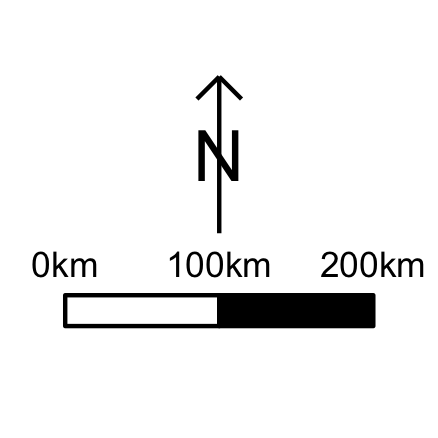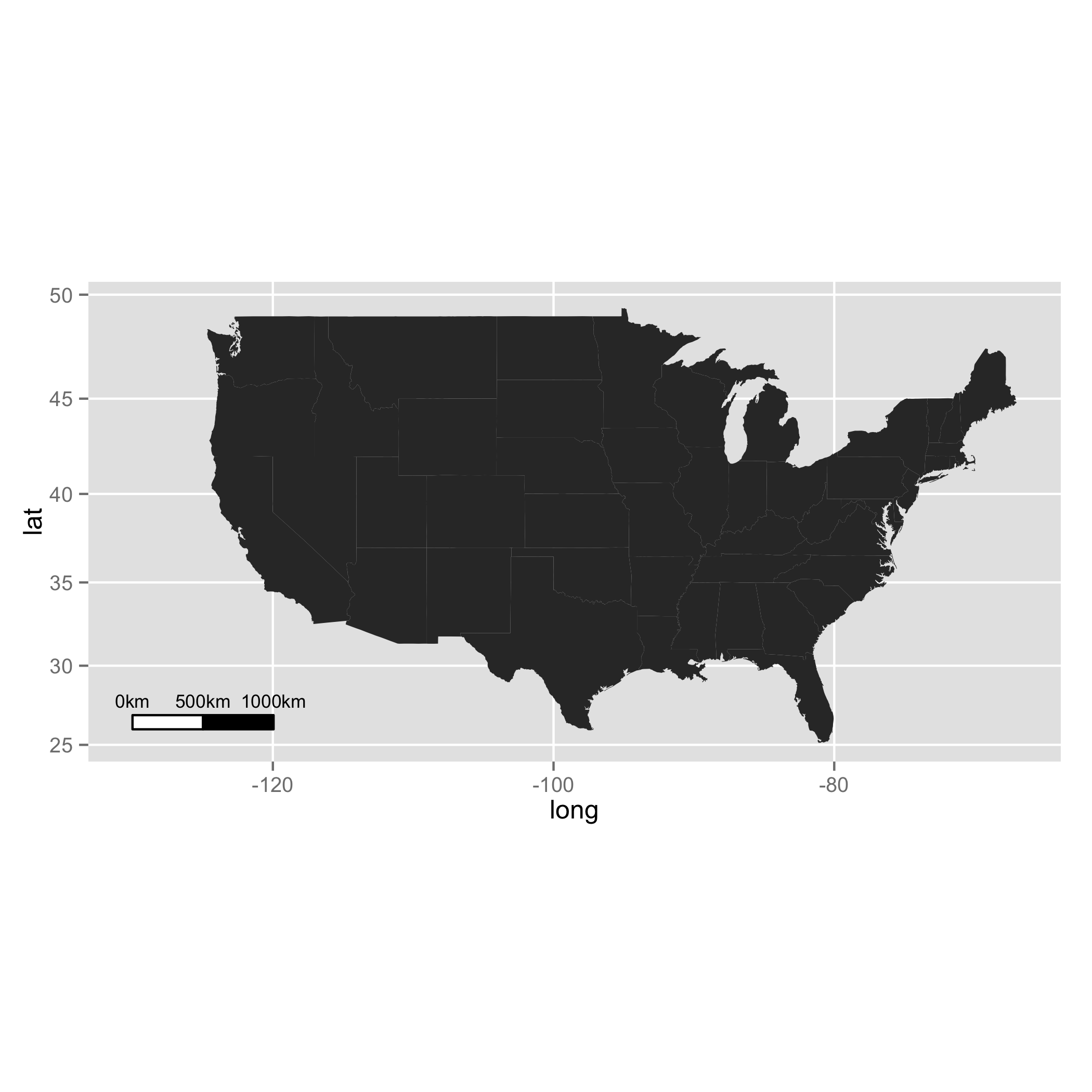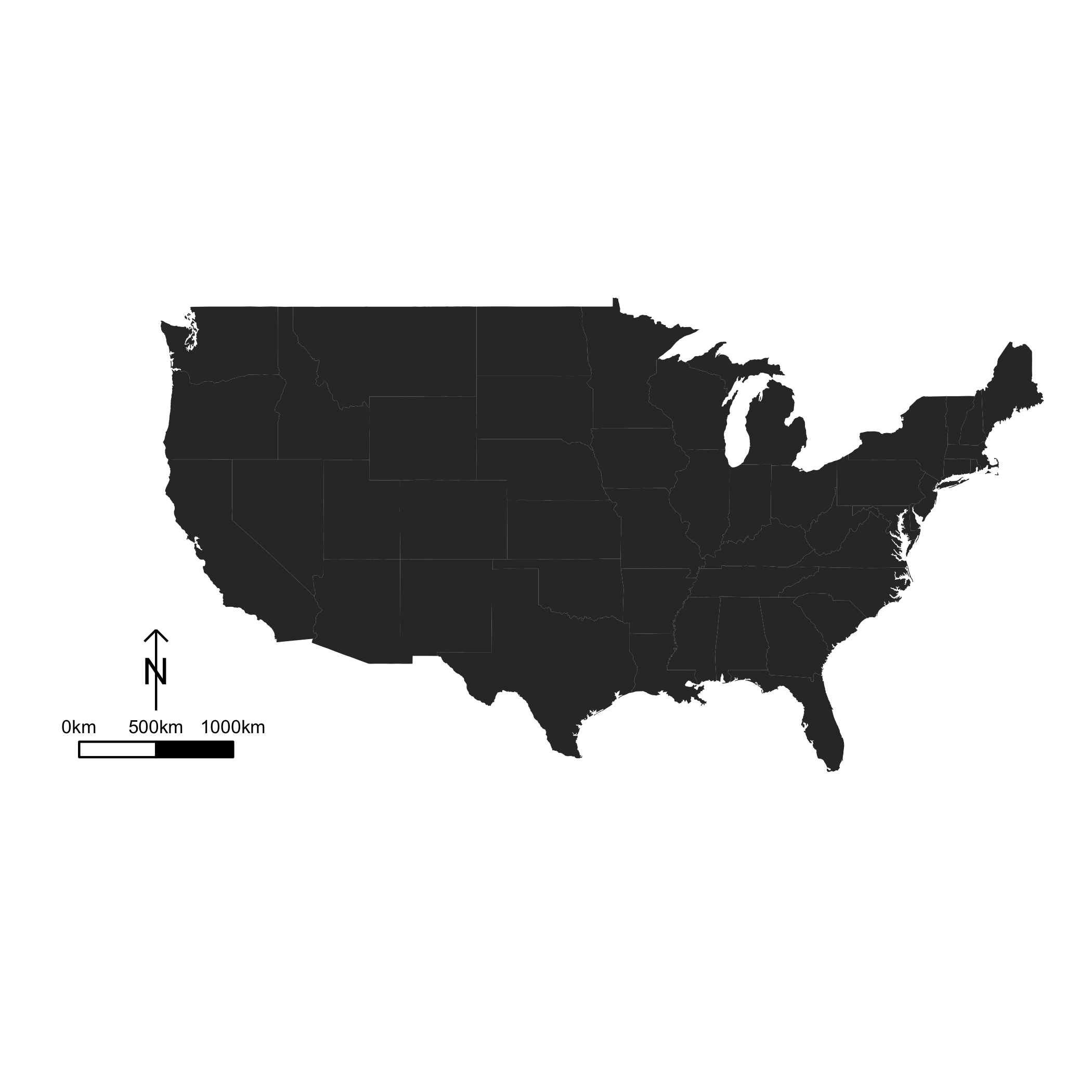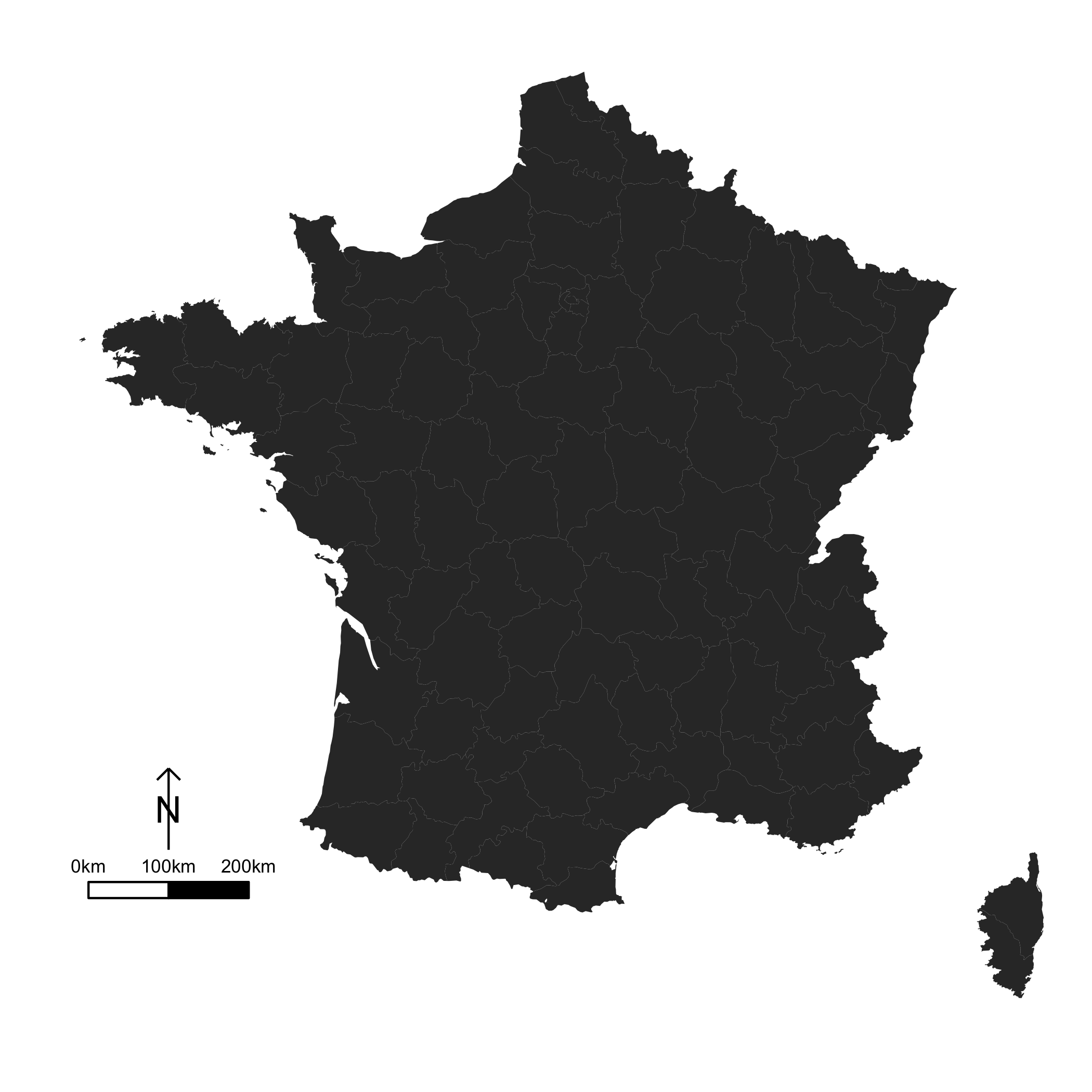After some research on the Internet, I gave up trying to find an R function to add a scale bar and a North arrow on a map, using ggplot().
So, I would like to present you what I have come up with.The idea is really basic : we create two polygons for the scale bar, we add some text above, and we draw an arrow. That’s it. The only tricky thing here, is the way to obtain the coordinates of each element. An easy solution is to use the gcDestination function, from the maptools package.

The functions are available on github. To install the package:


devtools::install_github("3wen/legendMap")


From the user’s point of vue, there is only one function to use (scaleBar), and a few arguments to pass. However, this function has some dependencies.

First, let us load some packages:

library(maps)
library(maptools)
library(ggplot2)
library(grid)

Then, we need a function to get the scale bar coordinates:

#
# Result #
#--------#
# Return a list whose elements are :
# 	- rectangle : a data.frame containing the coordinates to draw the first rectangle ;
# 	- rectangle2 : a data.frame containing the coordinates to draw the second rectangle ;
# 	- legend : a data.frame containing the coordinates of the legend texts, and the texts as well.
#
# Arguments : #
#-------------#
# lon, lat : longitude and latitude of the bottom left point of the first rectangle to draw ;
# distance_lon : length of each rectangle ;
# distance_lat : width of each rectangle ;
# distance_legend : distance between rectangles and legend texts ;
# dist_units : units of distance "km" (kilometers) (default), "nm" (nautical miles), "mi" (statute miles).
create_scale_bar <- function(lon,lat,distance_lon,distance_lat,distance_legend, dist_units = "km"){
# First rectangle
bottom_right <- gcDestination(lon = lon, lat = lat, bearing = 90, dist = distance_lon, dist.units = dist_units, model = "WGS84")

topLeft <- gcDestination(lon = lon, lat = lat, bearing = 0, dist = distance_lat, dist.units = dist_units, model = "WGS84")
rectangle <- cbind(lon=c(lon, lon, bottom_right[1,"long"], bottom_right[1,"long"], lon),
lat = c(lat, topLeft[1,"lat"], topLeft[1,"lat"],lat, lat))
rectangle <- data.frame(rectangle, stringsAsFactors = FALSE)

# Second rectangle t right of the first rectangle
bottom_right2 <- gcDestination(lon = lon, lat = lat, bearing = 90, dist = distance_lon*2, dist.units = dist_units, model = "WGS84")
rectangle2 <- cbind(lon = c(bottom_right[1,"long"], bottom_right[1,"long"], bottom_right2[1,"long"], bottom_right2[1,"long"], bottom_right[1,"long"]),
lat=c(lat, topLeft[1,"lat"], topLeft[1,"lat"], lat, lat))
rectangle2 <- data.frame(rectangle2, stringsAsFactors = FALSE)

# Now let's deal with the text
on_top <- gcDestination(lon = lon, lat = lat, bearing = 0, dist = distance_legend, dist.units = dist_units, model = "WGS84")
on_top2 <- on_top3 <- on_top
on_top2[1,"long"] <- bottom_right[1,"long"]
on_top3[1,"long"] <- bottom_right2[1,"long"]

legend <- rbind(on_top, on_top2, on_top3)
legend <- data.frame(cbind(legend, text = c(0, distance_lon, distance_lon*2)), stringsAsFactors = FALSE, row.names = NULL)
return(list(rectangle = rectangle, rectangle2 = rectangle2, legend = legend))
}


We also need a function to obtain the coordinates of the North arrow:

#
# Result #
#--------#
# Result #
#--------#
# Returns a list containing :
#	- res : coordinates to draw an arrow ;
#	- coordinates of the middle of the arrow (where the "N" will be plotted).
#
# Arguments : #
#-------------#
# scale_bar : result of create_scale_bar() ;
# length : desired length of the arrow ;
# distance : distance between legend rectangles and the bottom of the arrow ;
# dist_units : units of distance "km" (kilometers) (default), "nm" (nautical miles), "mi" (statute miles).
create_orientation_arrow <- function(scale_bar, length, distance = 1, dist_units = "km"){
lon <- scale_bar$rectangle2[1,1] lat <- scale_bar$rectangle2[1,2]

# Bottom point of the arrow
beg_point <- gcDestination(lon = lon, lat = lat, bearing = 0, dist = distance, dist.units = dist_units, model = "WGS84")
lon <- beg_point[1,"long"]
lat <- beg_point[1,"lat"]

# Let us create the endpoint
on_top <- gcDestination(lon = lon, lat = lat, bearing = 0, dist = length, dist.units = dist_units, model = "WGS84")

left_arrow <- gcDestination(lon = on_top[1,"long"], lat = on_top[1,"lat"], bearing = 225, dist = length/5, dist.units = dist_units, model = "WGS84")

right_arrow <- gcDestination(lon = on_top[1,"long"], lat = on_top[1,"lat"], bearing = 135, dist = length/5, dist.units = dist_units, model = "WGS84")

res <- rbind(
cbind(x = lon, y = lat, xend = on_top[1,"long"], yend = on_top[1,"lat"]),
cbind(x = left_arrow[1,"long"], y = left_arrow[1,"lat"], xend = on_top[1,"long"], yend = on_top[1,"lat"]),
cbind(x = right_arrow[1,"long"], y = right_arrow[1,"lat"], xend = on_top[1,"long"], yend = on_top[1,"lat"]))

res <- as.data.frame(res, stringsAsFactors = FALSE)

# Coordinates from which "N" will be plotted
coords_n <- cbind(x = lon, y = (lat + on_top[1,"lat"])/2)

return(list(res = res, coords_n = coords_n))
}


The last function enables the user to draw the elements:

#
# Result #
#--------#
# This function enables to draw a scale bar on a ggplot object, and optionally an orientation arrow #
# Arguments : #
#-------------#
# lon, lat : longitude and latitude of the bottom left point of the first rectangle to draw ;
# distance_lon : length of each rectangle ;
# distance_lat : width of each rectangle ;
# distance_legend : distance between rectangles and legend texts ;
# dist_units : units of distance "km" (kilometers) (by default), "nm" (nautical miles), "mi" (statute miles) ;
# rec_fill, rec2_fill : filling colour of the rectangles (default to white, and black, resp.);
# rec_colour, rec2_colour : colour of the rectangles (default to black for both);
# legend_colour : legend colour (default to black);
# legend_size : legend size (default to 3);
# orientation : (boolean) if TRUE (default), adds an orientation arrow to the plot ;
# arrow_length : length of the arrow (default to 500 km) ;
# arrow_distance : distance between the scale bar and the bottom of the arrow (default to 300 km) ;
# arrow_north_size : size of the "N" letter (default to 6).
scale_bar <- function(lon, lat, distance_lon, distance_lat, distance_legend, dist_unit = "km", rec_fill = "white", rec_colour = "black", rec2_fill = "black", rec2_colour = "black", legend_colour = "black", legend_size = 3, orientation = TRUE, arrow_length = 500, arrow_distance = 300, arrow_north_size = 6){
the_scale_bar <- create_scale_bar(lon = lon, lat = lat, distance_lon = distance_lon, distance_lat = distance_lat, distance_legend = distance_legend, dist_unit = dist_unit)
# First rectangle
rectangle1 <- geom_polygon(data = the_scale_bar$rectangle, aes(x = lon, y = lat), fill = rec_fill, colour = rec_colour) # Second rectangle rectangle2 <- geom_polygon(data = the_scale_bar$rectangle2, aes(x = lon, y = lat), fill = rec2_fill, colour = rec2_colour)

# Legend
scale_bar_legend <- annotate("text", label = paste(the_scale_bar$legend[,"text"], dist_unit, sep=""), x = the_scale_bar$legend[,"long"], y = the_scale_bar$legend[,"lat"], size = legend_size, colour = legend_colour) res <- list(rectangle1, rectangle2, scale_bar_legend) if(orientation){# Add an arrow pointing North coords_arrow <- create_orientation_arrow(scale_bar = the_scale_bar, length = arrow_length, distance = arrow_distance, dist_unit = dist_unit) arrow <- list(geom_segment(data = coords_arrow$res, aes(x = x, y = y, xend = xend, yend = yend)), annotate("text", label = "N", x = coords_arrow$coords_n[1,"x"], y = coords_arrow$coords_n[1,"y"], size = arrow_north_size, colour = "black"))
res <- c(res, arrow)
}
return(res)
}


Now, let’s play with this!

Let us draw the US map:

# United States map
usa_map <- map_data("state")
P <- ggplot() + geom_polygon(data = usa_map, aes(x = long, y = lat, group = group)) + coord_map()


If we want to add the scale bar only:

P + scale_bar(lon = -130, lat = 26,
distance_lon = 500, distance_lat = 100, distance_legend = 200,
dist_unit = "km", orientation = FALSE)US map using ggplot2 and scaleBar, without North arrow

Or if we want the scale bar and the North arrow:

P <- P + scale_bar(lon = -130, lat = 26,
distance_lon = 500, distance_lat = 100,
distance_legend = 200, dist_unit = "km")


To make it look more like a map:

P + theme(panel.grid.minor = element_line(colour = NA), panel.grid.minor = element_line(colour = NA),
panel.background = element_rect(fill = NA, colour = NA), axis.text.x = element_blank(),
axis.text.y = element_blank(), axis.ticks.x = element_blank(),
axis.ticks.y = element_blank(), axis.title = element_blank(),
rect = element_blank(),
plot.margin = unit(0 * c(-1.5, -1.5, -1.5, -1.5), "lines"))US map using ggplot2 and scaleBar

Another example, with France:

france_map <- map_data("france")
P_fr <- ggplot() + geom_polygon(data = france_map, aes(x = long, y = lat, group = group)) + coord_map()

# Let us add the scale bar
P_fr <- P_fr + scale_bar(lon = -5, lat = 42.5,
distance_lon = 100, distance_lat = 20,
distance_legend = 40, dist_unit = "km",
arrow_length = 100, arrow_distance = 60, arrow_north_size = 6)

# Modifying the theme a bit
P_fr + theme(panel.grid.minor = element_line(colour = NA), panel.grid.minor = element_line(colour = NA),
panel.background = element_rect(fill = NA, colour = NA), axis.text.x = element_blank(),
axis.text.y = element_blank(), axis.ticks.x = element_blank(),
axis.ticks.y = element_blank(), axis.title = element_blank(),
rect = element_blank(),
plot.margin = unit(0 * c(-1.5, -1.5, -1.5, -1.5), "lines"))France map using ggplot2 and scaleBar

## 26 thoughts on “Scale bar and North arrow on a ggplot2 map using R”

1.Saki Takahashi says:

Hello,

I just wanted to let you know that this was incredibly helpful – I’ve struggled with making good scalebars for a while now, and this is exactly what I needed! Merci beaucoup!

Cheers,
Saki

1.Ewen says:

Hi! You are welcome. Glad I could help!

2.ST says:

This looks fantastic, but when I try to plot it I get the message:
Error in eval(expr, envir, enclos) : object 'group' not found

 

Do you know why this happens and how it can be resolved?

1.Ewen says:

Hi,
I guess this is because there is no “group” column in your data frame.
P <- ggplot() + geom_polygon(data = usa.map, aes(x = long, y = lat, group = group))
The argument "group" is here because there are multiple polygons for each state, and since I want to join the points, I need to be careful with these polygons.
If you are using another map, from maps package, or a shapefile, you might find the function "fortify" from ggplot2 package very useful.

3.ST says:

Thanks for your response Ewen. I was using data that had been manipulated using the fortify function, sorry for not making that clear.
I seem to have resolved my issue. Previously, I had not explicitly stated data = spatial.df when building the layers in ggplot, i.e. I did geom_polygon(spatial.df, aes(...))
Doing so seems to resolve my problem.

 map <- ggplot() + geom_polygon(data = coast.df, aes(long, lat, group = group)) + geom_path(data = coast.df, aes(long, lat,group=group), colour = "white") + coord_map() #library(mapproj) map 

4.Sara says:

Hello!
First of all, thanks a lot for your code, I’ve been struggling with this problem for a while and I think this might save me! 🙂 However, when I try to add the scale bar, I get this warning:

Error in data.frame(cbind(legend, text = c(0, distanceLon, distanceLon * :
arguments imply differing number of rows: 0, 1
In addition: Warning message:
In cbind(legend, text = c(0, distanceLon, distanceLon * 2)) :
number of rows of result is not a multiple of vector length (arg 2)

Do you know how to solve this or what I might be doing wrong?

1.Ewen says:

Hello,

I’m sorry but I have no idea. Maybe if you could give me an example, I might be able to help you.

Regards.

5.David Oseguera Montiel says:

Hi Ewen,
Thank you very much for making and sharing a great work. I did not have a clue how to do it.
Regards,
David

6.Jess Allen says:

Can’t thank you enough – been trying to get this right for a good while now!
Code works beautifully!

Regards
Jess

7.Fernando Cagua says:

You are just awesome. Thanks a lot for sharing this code. Its very well done 🙂

1.Ewen says:

Thank you for using it!

8.Carolina says:

I think this is what I need, only, I’m working with GaussKruger data, what should I do (where should I change things to adjust this to my needs?

Thankyou!

1.Ewen says:

Hi, maybe you can try to change the choice of ellipsoide model in all calls to the gcDestination function. Or try to play with spTransform() and sp objects…

9.LGirl says:

Hi there, I have been looking for a way to do this for a long time! Many thanks. I am very new to R and was wondering, in order to create a scale bar on a map I am drawing, the first three sections where we are creating the scale bar… do I just paste in the code you have given into my console and then change the ‘function’ line (i.e., lon = xx, lon = xx, distancelon = xx… etc. etc.) but leave the rest as you have written? Will it automatically calculate the length from my map?

Many thanks

1.Ewen says:

Hi! You can copy/paste the functions in your console or your script file and then execute the code. From this moment, you will be able to use these functions. You don’t need to change them. What you need to do, is to change the values of the arguments when you call the function.

First create your ggplot map, and add the scale bar as an extra layer, using the “+” sign.
your_ggplot_object + scaleBar(lon = -130, lat = 26, distanceLon = 500, distanceLat = 100, distanceLegend = 200, dist.unit = "km")

On the example above, I call the “scaleBar” function, and I specify some values for the arguments. For instance, lon = -130, lat = 26 means I want the bottom left point of the rectangle to be at (-130,26). You need to define the other values of each argument according to what you want (there is a description of each argument in the header of the function).

10.Rafael says:

Great!

11.jjunju says:

I have tried our code but i get an error  Error: 'x' and 'units' must have length > 0

 

here is my code  m <- get_map(location =loc ,color = "color",source = "google",maptype = c("terrain"),zoom = Zoom)

 p<-ggmap(m)#,ylim=YL,xlim=XL)) #polygon rivers p<-p+ geom_path(aes(x=long,y=lat,group=group),fill="grey",size=.5,color="cadetblue",data=riv) #Waterways p<-p+geom_segment(size=2,data=boxes,aes(y = boxes$LATin, x = boxes$LONin, yend = boxes$LATph, xend = boxes$LONph),colour="black",alpha=0.5) #Intakes boxes\$shape=factor("intake") p<-p+geom_point(data=boxes, aes(x=LONin, y=LATin,shape=25), fill="lightblue", size=4)+scale_shape_identity() #Powerhouses p<-p+ geom_point(data=boxes, aes(x=LONph, y=LATph,shape=22), fill="red", size=3) #Labels p<-p+ geom_text(size=2.8,fontface="bold",hjust=-0.2,data = boxes, aes(x=LONph,y=LATph,label = paste(mw, "MW",sep=""))) p<-p+ geom_text(size=2.8,fontface="bold",colour="blue",hjust=-1,angle=90,data = boxes, aes(x=LONin,y=LATin,label = paste("[",IDn,"]n",sep=""))) print(p) 

#scale bar source('~/Documents/DATA/Dev/R/HydroSearch/RAW/LakeGeorge1k/scale.bar.r') (q <- p + scaleBar(lon = 29.5, lat = -0.6, distanceLon = 20, distanceLat = 10, distanceLegend = 20, dist.unit = "km")

1.Ewen says:

Hi,

It’s hard to be sure with your code, since it is not reproducible. But my guess is that you are trying to draw the scale bar outside the range of the Google map coordinates.
Try changing the lon and lat parameters, and/or the distanceLon and distanceLat. 🙂

12.James says:

Hey, thanks a million.. I wonder if it would be easy to add a text.colour option to set the legend text colour as required?

1.Ewen Gallic says:

Hi James, do you mean in the package that is uploaded on Github?

13.Johan says:

Thanks for an awesome code! I currently work with rather small maps and would like to change the units from kilometers to meters. Just changing the “km” to “m” but that doesn’t seem to work. Any suggestions?

1.Ewen Gallic says:

Hello! Thanks! You the parameter dist_units allows you to change from km to nautical miles or statute miles. If you work with small maps, just play with the three following parameters: distance_lon, distance_lat, and distance_legend . Just keep in mind that the values are expressed in kilometers, so pick smaller values!

14.Eleni Petrou says:

Thank you for sharing your code! It worked wonderfully!

15.Federico says:

Hi!

First of all, congrats and thank you for this very useful code! I have one question though, I am having trouble adding the scale bar every time I create a faceted ggplot.

this is the error message I get: “Error: Aesthetics must be either length 1 or the same as the data (9): label, colour, size”

this an example of the code that is giving me trouble:

map1 +
geom_point(data=Effort_DB,
aes(y=Latitud,x=Longitud),
fill=”red”,color=”grey50″,size=2,shape=21,alpha=.6) +
geom_point(data=Localidades,aes(y=Latitud,x=Longitud),
shape=17,color=”black”,size=2) +
geom_text(data=Localidades,aes(y=Latitud,x=Longitud,label=Localidad),
vjust=0,hjust=-.1,check_overlap=F,angle=90,size=4.5) +
theme(strip.text=element_text(size=14,face=”bold”)) +
scaleBar(lon=-53.85,lat=-35.87,distanceLon=30,distanceLat=5,distanceLegend=10,dist.unit=”km”,legend.size=4.3) +
facet_grid(.~Arte)

Clearly the problem is realted to faceting, but I can’t figure out what is wrong.

Thanks in advance for your help 🙂

16.Kris says:

Thank you for this code! It save me!!

17.Brittany says:

Hi Ewen,
Thank you so much for creating this! It works perfectly for my larger scale maps, however, I’m having trouble with the text on a smaller scale map. The text portion of the scale bar is floating too far above the polygons rather than neatly on top. Is there a way to change this?